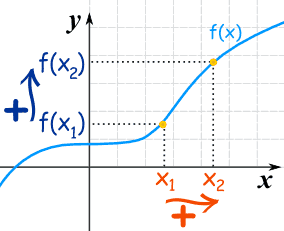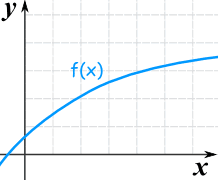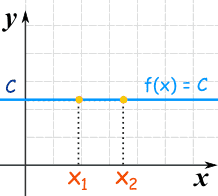# Increasing and Decreasing Functions

## Increasing Functions

A function is "increasing" when the y-value increases as the x-value increases, like this:It is easy to see that y=f(x) tends to go up as it goes along.

### Flat?

What about that flat bit near the start? Is that OK?

• Yes, it is OK when we say the function is Increasing
• But it is not OK if we say the function is Strictly Increasing (no flatness allowed)

### Using Algebra

What if we can't plot the graph to see if it is increasing? In that case we need a definition using algebra.

For a function y=f(x):

 when x1 < x2 then f(x1) ≤ f(x2) Increasing when x1 < x2 then f(x1) < f(x2) Strictly Increasing

That has to be true for any x1, x2, not just some nice ones we might choose.

The important parts are the < and signs ... remember where they go!

### An Example:This is also an increasing function even though the rate of increase reduces

### For An Interval

Usually we are only interested in some interval, like this one:This function is increasing for the interval shown
(it may be increasing or decreasing elsewhere)

## Decreasing Functions

The y-value decreases as the x-value increases:For a function y=f(x):

 when x1 < x2 then f(x1) ≥ f(x2) Decreasing when x1 < x2 then f(x1) > f(x2) Strictly Decreasing

Notice that f(x1) is now larger than (or equal to) f(x2).

## An Example

Let us try to find where a function is increasing or decreasing.

### Example: f(x) = x3−4x, for x in the interval [−1,2]

Let us plot it, including the interval [−1,2]:Starting from −1 (the beginning of the interval [−1,2]):

• at x = −1 the function is decreasing,
• it continues to decrease until about 1.2
• it then increases from there, past x = 2

Without exact analysis we cannot pinpoint where the curve turns from decreasing to increasing, so let us just say:

Within the interval [−1,2]:

• the curve decreases in the interval [−1, approx 1.2]
• the curve increases in the interval [approx 1.2, 2]

## Constant Functions

A Constant Function is a horizontal line:## Lines

In fact lines are either increasing, decreasing, or constant.

The equation of a line is:

y = mx + bThe slope m tells us if the function is increasing, decreasing or constant:

 m < 0 decreasing m = 0 constant m > 0 increasing

## One-to-One

Strictly Increasing (and Strictly Decreasing) functions have a special property called "injective" or "one-to-one" which simply means we never get the same "y" value twice.General Function"Injective" (one-to-one)

Why is this useful? Because Injective Functions can be reversed!

We can go from a "y" value back to an "x" value (which we can't do when there is more than one possible "x" value).

Read Injective, Surjective and Bijective to find out more.